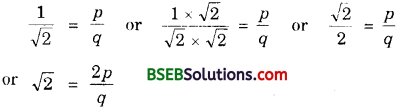# HSSlive: Plus One & Plus Two Notes & Solutions for Kerala State Board

## BSEB Class 10 Maths Chapter 1 Real Numbers Ex 1.3 Textbook Solutions PDF: Download Bihar Board STD 10th Maths Chapter 1 Real Numbers Ex 1.3 Book AnswersBSEB Class 10 Maths Chapter 1 Real Numbers Ex 1.3 Textbook Solutions PDF: Download Bihar Board STD 10th Maths Chapter 1 Real Numbers Ex 1.3 Book Answers

BSEB Class 10th Maths Chapter 1 Real Numbers Ex 1.3 Textbooks Solutions and answers for students are now available in pdf format. Bihar Board Class 10th Maths Chapter 1 Real Numbers Ex 1.3 Book answers and solutions are one of the most important study materials for any student. The Bihar Board Class 10th Maths Chapter 1 Real Numbers Ex 1.3 books are published by the Bihar Board Publishers. These Bihar Board Class 10th Maths Chapter 1 Real Numbers Ex 1.3 textbooks are prepared by a group of expert faculty members. Students can download these BSEB STD 10th Maths Chapter 1 Real Numbers Ex 1.3 book solutions pdf online from this page.

## Bihar Board Class 10th Maths Chapter 1 Real Numbers Ex 1.3 Books Solutions

 Board BSEB Materials Textbook Solutions/Guide Format DOC/PDF Class 10th Subject Maths Chapter 1 Real Numbers Ex 1.3 Chapters All Provider Hsslive

## How to download Bihar Board Class 10th Maths Chapter 1 Real Numbers Ex 1.3 Textbook Solutions Answers PDF Online?

2. Click on the Bihar Board Class 10th Maths Chapter 1 Real Numbers Ex 1.3 Answers.
3. Look for your Bihar Board STD 10th Maths Chapter 1 Real Numbers Ex 1.3 Textbooks PDF.
4. Now download or read the Bihar Board Class 10th Maths Chapter 1 Real Numbers Ex 1.3 Textbook Solutions for PDF Free.

## BSEB Class 10th Maths Chapter 1 Real Numbers Ex 1.3 Textbooks Solutions with Answer PDF Download

Find below the list of all BSEB Class 10th Maths Chapter 1 Real Numbers Ex 1.3 Textbook Solutions for PDF’s for you to download and prepare for the upcoming exams:

## BSEB Bihar Board Class 10th Maths Solutions Chapter 1 Real Numbers Ex 1.3

Question 1.
Prove that 5‾√ is irrational. [CBSE, Delhi 2009]
Solution:
Let us assume, to the contrary, that 5‾√ is rational.
Squaring on both sides, we get
5 = 𝑎2𝑏2 or 5b2 = a2 ………………. (1)
This shows that a2 is divisible by 5.
It follows that a is divisible by 5. …………………. (2)
So, a = 5m for some integer m.
Substituting a = 5m in (1), we get
5b2 = (5m)2 = 25m2
or b2 = 5m2 or b2 is divisible by 5
and hence b is divisible by 5.
From (2) and (3), we can conclude that 5 is a common factor of both a and b.
But this contradicts our supposition that a and b are coprime.
Hence, 5‾√ is irrational.

Question 2.
Prove that 3 + 25‾√ is irrational.
Solution:
Let us assume, to the contrary, that 3 + 25‾√ is a rational number.
Now, let 3 + 25‾√ = 𝑎𝑏, where a and b are coprime and b ≠ 0.
So, 25‾√ = 𝑎𝑏 – 3 or 5‾√ = 𝑎2𝑏 – 32
Since a and b are integers, therefore
𝑎2𝑏 – 32 is a rational number.
∴ 5‾√ is an irrational number.
But 5‾√ is an irrational number.
This shows that our assumption is incorrect.
So, 3 + 25‾√ is an irrational number.

Question 3.
Prove that the following are irrationals:

1. 12√
2. 75‾√
3. 6 + 2‾√

Solution:
1. Let us assume, to the contrary, that 12√ is rational.
That is, we can find co-prime integers p and q (≠ 0) such thatSince p and q are integers, 2𝑝𝑞 is rational, and so 2‾√ is rational.
But this contradicts the fact that 2‾√ is irrational.
So, we conclude that 12√ is irrational.

2. Let us assume, to the contrary, that 75‾√ is rational.
That is, we can find co-prime integers p and q (≠ 0) such that 75‾√ = 𝑝𝑞.
So, 5‾√ = 𝑝7𝑞.
Since p and q are integers, 𝑝7𝑞 is rational and so is 5‾√.
But this contradicts the fact that 5‾√ is irrational.
So, we conclude that 75‾√ is irrational.

3. Let us assume, to the contrary, that 6 + 2‾√ is rational.
That is, we can find integers p and q (≠ 0) such that
6 + 2‾√ = 𝑝𝑞 or 6 – 𝑝𝑞 = 2‾√
or 2‾√ = 6 – 𝑝𝑞
Since p and q are integers, we get 6 – 𝑝𝑞 is rational, and so 2‾√ is rational.
But this contradicts the fact that 2‾√ is irrational.
So, we conclude that 6 + 2‾√ is irrational.

## Bihar Board Class 10th Maths Chapter 1 Real Numbers Ex 1.3 Textbooks for Exam Preparations

Bihar Board Class 10th Maths Chapter 1 Real Numbers Ex 1.3 Textbook Solutions can be of great help in your Bihar Board Class 10th Maths Chapter 1 Real Numbers Ex 1.3 exam preparation. The BSEB STD 10th Maths Chapter 1 Real Numbers Ex 1.3 Textbooks study material, used with the English medium textbooks, can help you complete the entire Class 10th Maths Chapter 1 Real Numbers Ex 1.3 Books State Board syllabus with maximum efficiency.

## FAQs Regarding Bihar Board Class 10th Maths Chapter 1 Real Numbers Ex 1.3 Textbook Solutions

#### Can we get a Bihar Board Book PDF for all Classes?

Yes you can get Bihar Board Text Book PDF for all classes using the links provided in the above article.

## Important Terms

Bihar Board Class 10th Maths Chapter 1 Real Numbers Ex 1.3, BSEB Class 10th Maths Chapter 1 Real Numbers Ex 1.3 Textbooks, Bihar Board Class 10th Maths Chapter 1 Real Numbers Ex 1.3, Bihar Board Class 10th Maths Chapter 1 Real Numbers Ex 1.3 Textbook solutions, BSEB Class 10th Maths Chapter 1 Real Numbers Ex 1.3 Textbooks Solutions, Bihar Board STD 10th Maths Chapter 1 Real Numbers Ex 1.3, BSEB STD 10th Maths Chapter 1 Real Numbers Ex 1.3 Textbooks, Bihar Board STD 10th Maths Chapter 1 Real Numbers Ex 1.3, Bihar Board STD 10th Maths Chapter 1 Real Numbers Ex 1.3 Textbook solutions, BSEB STD 10th Maths Chapter 1 Real Numbers Ex 1.3 Textbooks Solutions,
Share: